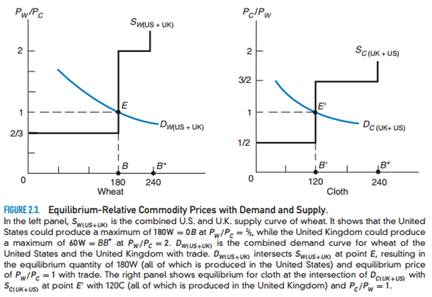### Create an Account

Home / Questions / a What would be the equilibrium-relative commodity price of wheat if DW US UK shifted up ...

# a What would be the equilibrium-relative commodity price of wheat if DW US UK shifted up by one third in the left panel of Figure 2 3 How much wheat and cloth would the United States and the

a) What would be the equilibrium-relative commodity price of wheat if DW (US+UK) shifted up by one-third in the left panel of Figure 2.3? How much wheat and cloth would the United States and the United Kingdom then produce?

(b) What does the answer to part (a) imply for DC(UK+US) in the right panel of Figure 2.3?Jul 15 2020 View more View LessSubscribe To Get Solution